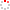IMR OpenIR
 The modified KdV equation with variable coefficients: Exact uni/bi-variable travelling wave-like solutions; The modified KdV equation with variable coefficients: Exact uni/bi-variable travelling wave-like solutions Z. Y. Yan 2008 ; 2008 Source Publication Applied Mathematics and Computation; Applied Mathematics and ComputationISSN 0096-3003 ; 0096-3003 Volume 203Issue:1Pages:106-112 Abstract In this paper, the modified Korteweg-de Vries (mKdV) equation with variable coefficients (vc-mKdV equation) is investigated via two kinds of approaches and symbolic computation. On the one hand, we firstly reduce the vc-mKdV equation to a second-order nonlinear nonhomogeneous ODE using travelling wave-like similarity transformation. And then we obtain its many types of exact fractional solutions with one travelling wave-like variable by applying some fractional transformations to the obtained nonlinear ODE. On the other hand, we reduce the vc-mKdV equation to two nonlinear PDEs with variable coefficients using the anti-tangent and anti-hypertangent function transformations, respectively. And then we given its many types of exact solutions with two different travelling wave-like variables by studying the obtained nonlinear PDE with variable coefficients. (c) 2008 Elsevier Inc. All rights reserved.; In this paper, the modified Korteweg-de Vries (mKdV) equation with variable coefficients (vc-mKdV equation) is investigated via two kinds of approaches and symbolic computation. On the one hand, we firstly reduce the vc-mKdV equation to a second-order nonlinear nonhomogeneous ODE using travelling wave-like similarity transformation. And then we obtain its many types of exact fractional solutions with one travelling wave-like variable by applying some fractional transformations to the obtained nonlinear ODE. On the other hand, we reduce the vc-mKdV equation to two nonlinear PDEs with variable coefficients using the anti-tangent and anti-hypertangent function transformations, respectively. And then we given its many types of exact solutions with two different travelling wave-like variables by studying the obtained nonlinear PDE with variable coefficients. (c) 2008 Elsevier Inc. All rights reserved. description.department [yan, zhenya] chinese acad sci, inst syst sci, key lab math mechanizat, amss, beijing 100080, peoples r china. [yan, zhenya] chinese acad sci, int ctr mat phys, shenyang 110016, peoples r china.;yan, zy (reprint author), chinese acad sci, inst syst sci, key lab math mechanizat, amss, beijing 100080, peoples r china;zyyan@mmrc.iss.ac.cn ; [yan, zhenya] chinese acad sci, inst syst sci, key lab math mechanizat, amss, beijing 100080, peoples r china. [yan, zhenya] chinese acad sci, int ctr mat phys, shenyang 110016, peoples r china.;yan, zy (reprint author), chinese acad sci, inst syst sci, key lab math mechanizat, amss, beijing 100080, peoples r china;zyyan@mmrc.iss.ac.cn Keyword Modified Korteweg-de Vries ( Mkdv) Modified Korteweg-de Vries ( Mkdv) Equation With Variable Coefficients Equation With Variable Coefficients Exact Solutions Exact Solutions De-vries Equation De-vries Equation Elliptic Function Solutions Elliptic Function Solutions Nonlinear Nonlinear Schrodinger-equation Schrodinger-equation Differential-equations Differential-equations Soliton Soliton URL 查看原文 ; 查看原文 WOS ID WOS:000258832700014 ; WOS:000258832700014 Citation statistics Cited Times:12[WOS]   [WOS Record]     [Related Records in WOS] Document Type 期刊论文 Identifier http://ir.imr.ac.cn/handle/321006/33265 Collection 中国科学院金属研究所 Recommended CitationGB/T 7714 Z. Y. Yan. The modified KdV equation with variable coefficients: Exact uni/bi-variable travelling wave-like solutions, The modified KdV equation with variable coefficients: Exact uni/bi-variable travelling wave-like solutions[J]. Applied Mathematics and Computation, Applied Mathematics and Computation,2008, 2008,203, 203(1):106-112, 106-112. APA Z. Y. Yan.(2008).The modified KdV equation with variable coefficients: Exact uni/bi-variable travelling wave-like solutions.Applied Mathematics and Computation,203(1),106-112. MLA Z. Y. Yan."The modified KdV equation with variable coefficients: Exact uni/bi-variable travelling wave-like solutions".Applied Mathematics and Computation 203.1(2008):106-112.
 Files in This Item: File Name/Size DocType Version Access License 619.pdf（184KB） 开放获取 --
 Related Services Recommend this item Bookmark Usage statistics Export to Endnote Google Scholar Similar articles in Google Scholar [Z. Y. Yan]'s Articles Baidu academic Similar articles in Baidu academic [Z. Y. Yan]'s Articles Bing Scholar Similar articles in Bing Scholar [Z. Y. Yan]'s Articles Terms of Use No data! Social Bookmark/Share